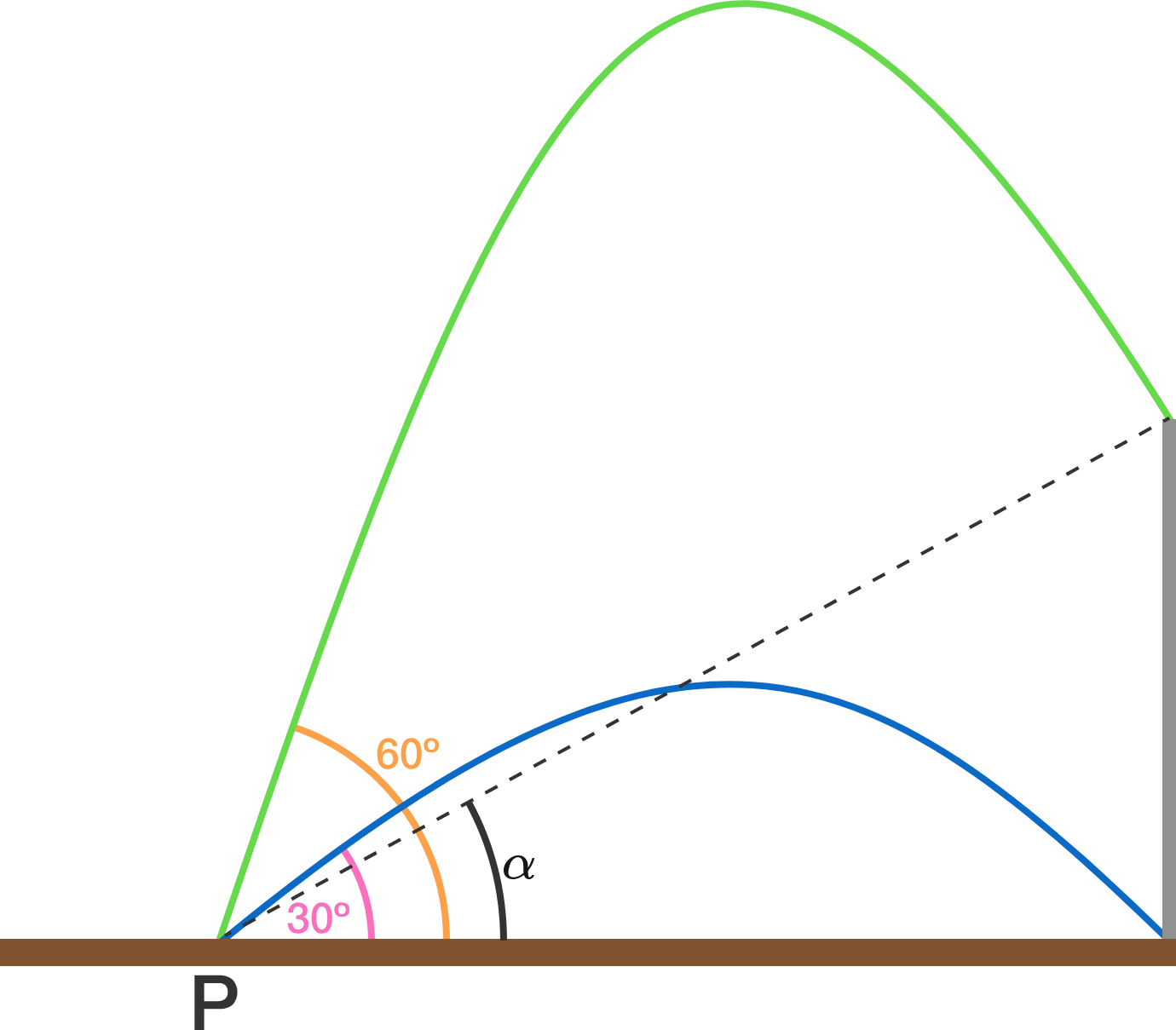# Not enough information!

There is a vertical pole perpendicular to the horizontal plane. From point $P$ on the plane, 2 projectiles are fired simultaneously at different velocities. The first projectile is fired at an angle of $30^{\circ}$ and it hits the foot of the pole. The second projectile is fired at an angle of $60^{\circ}$ and it hits the top of the pole.It is further known that the projectiles hit the pole at the same time.

If the angle subtended by the pole from $P$ is $\alpha$, find $\tan \alpha$ to 3 decimal places.


Details and Assumptions:

• Air resistance is negligible.
• A gravitational pull is present.
×

Problem Loading...

Note Loading...

Set Loading...